International
Tables for
Crystallography
Volume B
Reciprocal space
Edited by U. Shmueli

International Tables for Crystallography (2006). Vol. B, ch. 3.3, pp. 368-370   | 1 | 2 |

## Section 3.3.1.3.5. Windowing and perspective

R. Diamonda*

aMRC Laboratory of Molecular Biology, Hills Road, Cambridge CB2 2QH, England
Correspondence e-mail: rd10@cam.ac.uk

#### 3.3.1.3.5. Windowing and perspective

| top | pdf |

It is necessary at this point to relate the discussion to the axial system inherent in the graphics device employed. One common system adopts X horizontal and to the right when viewing the screen, Y vertically upwards in the plane of the screen, and Z normal to X and Y with +Z into the screen. This is, unfortunately, a left-handed system in that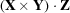is negative. Since it is usual in crystallographic work to use right-handed axial systems it is necessary to incorporate a matrix of the form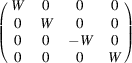either as the left-most factor in the matrix T or as the right-most factor in the windowing transformation U (see Section 3.3.1.3.1). The latter choice is to be preferred and is adopted here. The former choice leads to complications if transformations in display space will be required. Display-space coordinates are necessarily referred to this axial system.

Let L, R, T, B, N and F be the left, right, top, bottom, near and far boundaries of the windowed volume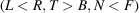, S be the Z coordinate of the screen, and C, D and E be the coordinates of the observer's eye position, all ten of these parameters being referred to the origin of display space as origin, which may be anywhere in relation to the hardware. L, R, T and B are to be evaluated in the screen plane. All ten parameters may be referred to their own fourth coordinate, V, meaning that the point (X, Y, Z, W) in display space will be on the left boundary of the picture if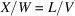when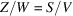. V may be freely chosen so that all eleven quantities and all elements of U suit the word length of the machine. These relationships are illustrated in Fig. 3.3.1.1.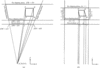Figure 3.3.1.1 | top | pdf |The relationship between display-space coordinates (X, Y, Z, W) and picture-space coordinates (x, y, z, w) derived from them by the window transformation, U. (a) Display space (in X, Z projection) showing a square object P, Q, R, S for display viewed from the position (C, D, E, V). The bold trapezium is the window (volume) and the bold line is the viewport portion of the screen. The points P, Q, R and S must be plotted at p, q, r and s to give the correct impression of the object. (b) Picture space (in x, z projection). The window is mapped to a rectangle and all sight lines are parallel to the z axis, but the object P, Q, R, S is no longer square. The distribution of p, q, r and s is identical in the two cases. Note that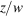values are not linear on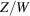, and that the origin of picture space arises at the midpoint of the near clipping plane, regardless of the location of the origin of display space. The figure is accurately to scale for coincident viewport positions. The words `Left clipping plane', if part of the scene in display space, would currently be obscured, but would come into view if the eye moved to the right, increasing C, as the left clipping plane would pivot about the point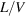in the screen plane.

Since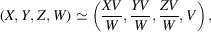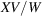is a display-space coordinate on the same scale as the window parameters. This must be plotted on the screen at an X coordinate (on the scale of the window parameters) which is the weighted mean ofand C, the weights being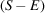and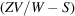, respectively. This provides perspective because the weighted mean is at the point where the straight line from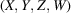to the eye intersects the screen. This then has to be mapped into the L-to-R interval, so that picture-space coordinates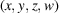are given by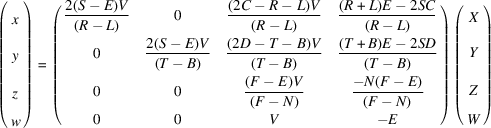which provides for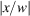and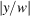to be unity on the picture boundaries, which is usually a requirement of the clipping hardware, and for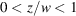, zero being for the near-plane boundary. Even thoughis not linear on, straight lines and planes in display space transform to straight lines and planes in picture space, the non-linearity affecting only distances. Thus vector-drawing machines are not disadvantaged by the introduction of perspective.

Note that the dimensionality of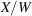must equal that of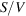and that this may be regarded as length or as a pure number, but that in either case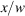is dimensionless, consistent with the stipulation that the picture boundaries be defined by the pure number ±1.

The above matrix is U and is suited to left-handed hardware systems. Note that only the last column of U (the translational part) is sensitive to the location of the origin of display space and that if the eye is on the normal to the picture centre then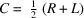,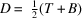and simplifications result. If C, D and E can be continuously monitored then dynamic parallax as well as perspective may be obtained (Diamond et al., 1982).

If data space is referred to right-handed axes, the viewing transformation T involves only proper rotations and the hardware uses a left-handed axial system then elements in the third column of U should be negated, as explained in the opening paragraph.

To provide for orthographic projection, multiply every element of U by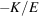and then let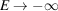, choosing some positive K to suit the word length of the machine [see Section 3.3.1.1.2(iii)]. The result is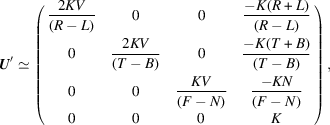which is the orthographic window.

It may be convenient in some applications to separate the functions of windowing and the application of perspective, and to write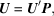where U and U′ are as above and P is a perspective transformation given by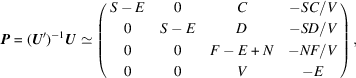which involves F and N but not R, L, T or B. In this form the action of P may be thought of as compressing distant parts of display space prior to an orthographic projection by U′ into picture space.

Other factorizations of U are possible, for example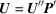with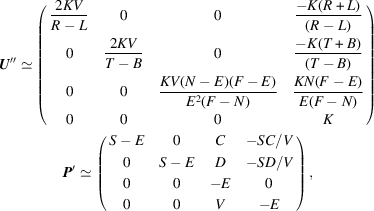which renders P′ independent of all six boundary planes, but U″ is no longer independent of E. It is not possible to factorize U so that the left factor is a function only of the boundary planes and the right factor a function only of eye and screen positions.

Note that as,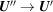, P and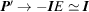.

### References

Diamond, R., Wynn, A., Thomsen, K. & Turner, J. (1982). Three-dimensional perception for one-eyed guys, or, the use of dynamic parallax. In Computational crystallography, edited by D. Sayre, pp. 286–293. Oxford University Press.Google Scholar